Question

# Butane gas of density = 2.5567 kg/m^3 flows at speed v1 in a horizontal pipe with...

Butane gas of density = 2.5567 kg/m^3 flows at speed v1 in a horizontal pipe with cross sectional area A1 = 0.0475 m^2 which widens to a larger still horizontal section of area A2 = 0.0665 m^2 in which it flows at speed v2. If the pressure difference between the two sections is measured accurately to be p2-p1 = 276.1236 Pa, Find (a) speed v1, and (b) the volume flow rate of the gas.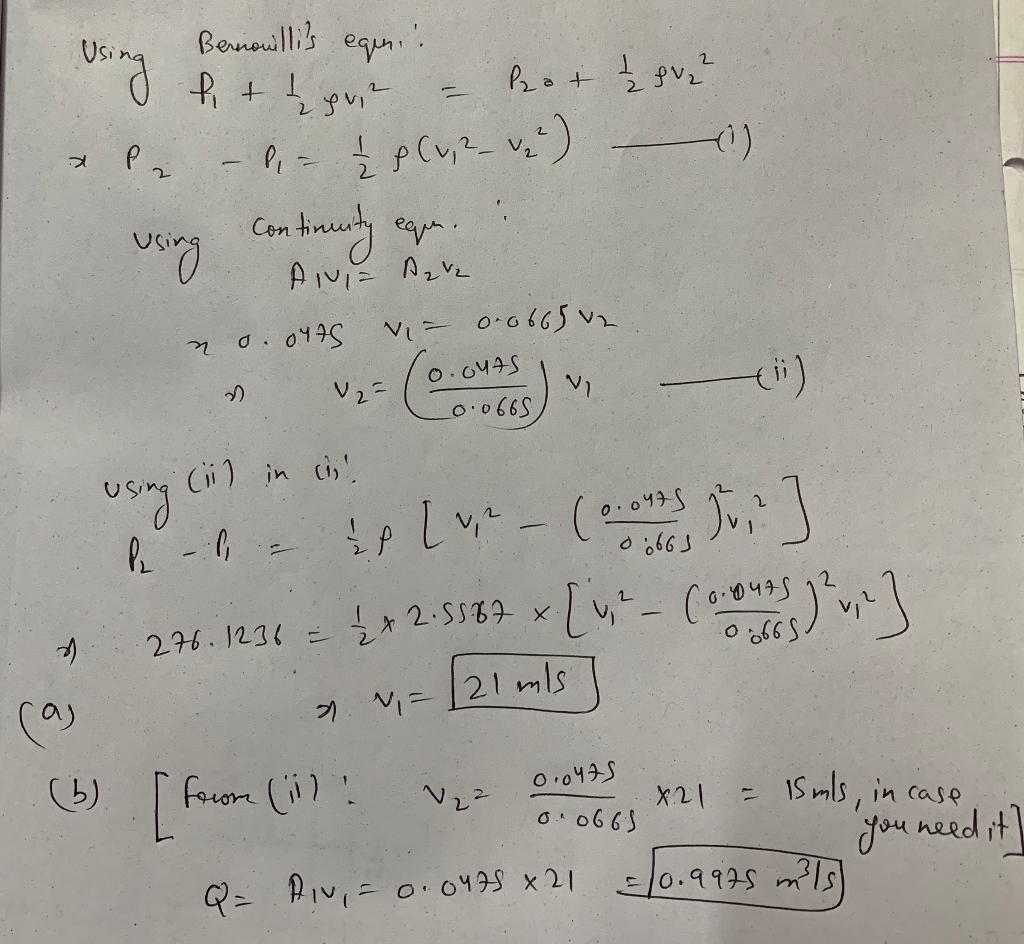Kindly upvote:)

#### Earn Coins

Coins can be redeemed for fabulous gifts.

Similar Homework Help Questions
• ### A venturi meter is a device for measuring the speed of a fluid within a pipe....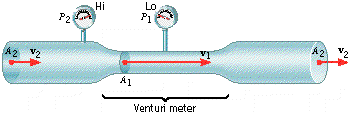A venturi meter is a device for measuring the speed of a fluid within a pipe. The drawing shows a gas flowing at a speed v2 through a horizontal section of pipe whose cross-sectional area A2 = 0.0700 m2. The gas has a density of ϝ = 1.30 kg/m3. The Venturi meter has a cross-sectional area of A1 = 0.0300 m2 and has been substituted for a section of the larger pipe. The pressure difference between the two sections is...

• ### A Venturi meter is a device for measuring the speed of a fluid within a pipe....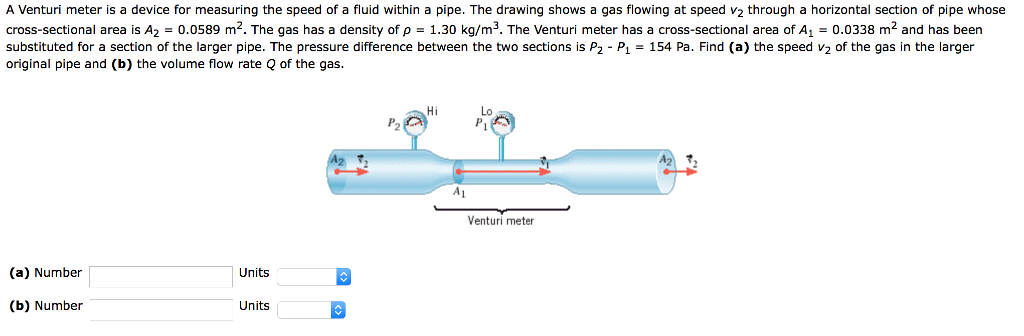A Venturi meter is a device for measuring the speed of a fluid within a pipe. The drawing shows a gas flowing at speed v2 through a horizontal section of pipe whose cross-sectional area is A2-0.0589 m2 The gas has a density of ρ-1.30 kg m3 The Venturi meter has a cross sectiona area of .ם 33m and has been substituted for a section of the larger pipe. The pressure difference between the two sections is P2 P1 154 Pa....

• ### show all work Water flows at v1 = 0.636 m/s through a pipe with cross-sectional area...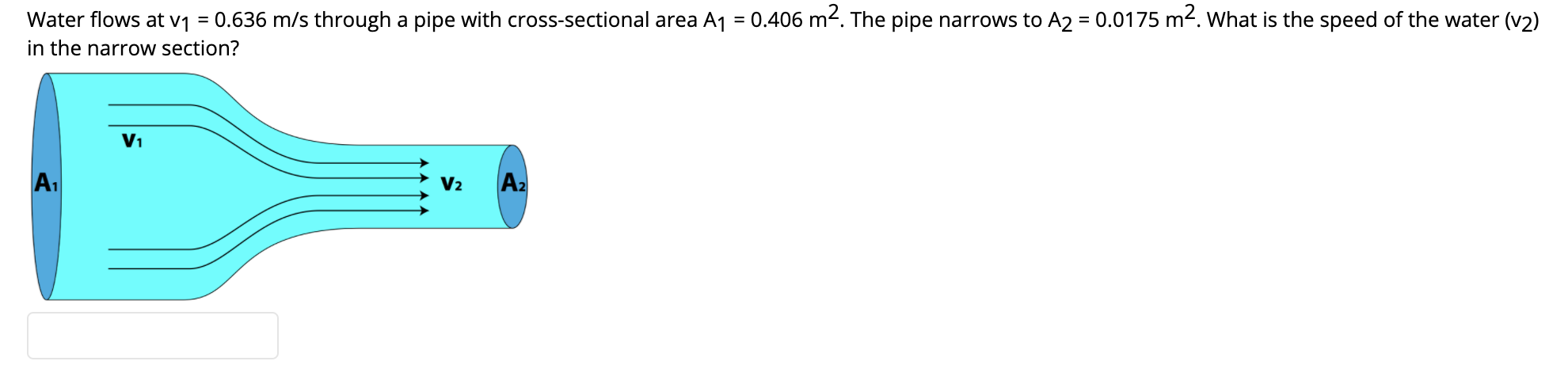show all work Water flows at v1 = 0.636 m/s through a pipe with cross-sectional area A1 = 0.406 m². The pipe narrows to A2 = 0.0175 m2. What is the speed of the water (v2) in the narrow section?

• ### Water flows with speed v1 inside a long pipe and exits from the right into the...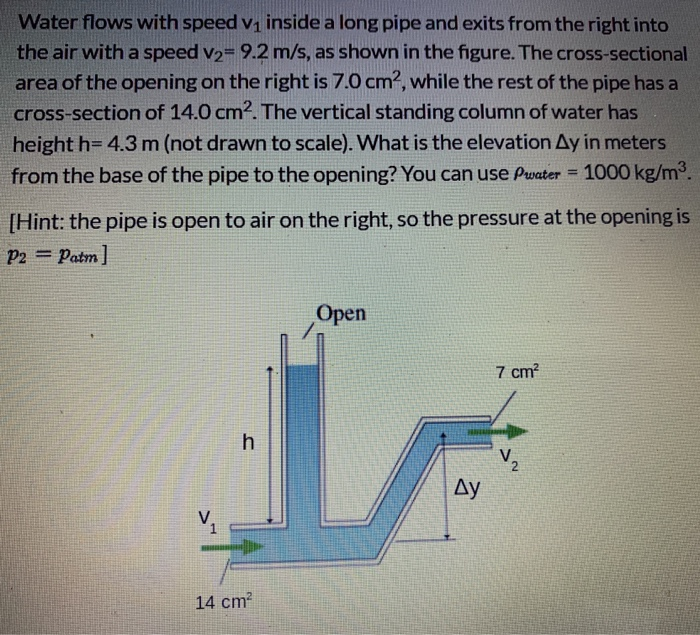Water flows with speed v1 inside a long pipe and exits from the right into the air with a speed v2= 9.2 m/s, as shown in the figure. The cross-sectional area of the opening on the right is 7.0 cm2, while the rest of the pipe has a cross-section of 14.0 cm2. The vertical standing column of water has height h= 4.3 m (not drawn to scale). What is the elevation Ay in meters from the base of the pipe...

• ### Water flows with speed v1 inside a long pipe and exits from the right into the...

Water flows with speed v1 inside a long pipe and exits from the right into the air with a speed v2= 6.8m/s. The cross-sectional area of the opening on the right is 6 cm^2, while the rest of the pipe has a cross-section of 12 cm^2. The vertical standing column of water has height of 4.8 m. What is the elevation in meters from the base of the pipe to the opening. Pwater= 1000 kg/m^3

• ### Question 3 1 pts Water flows with speed v1 inside a long pipe and exits from...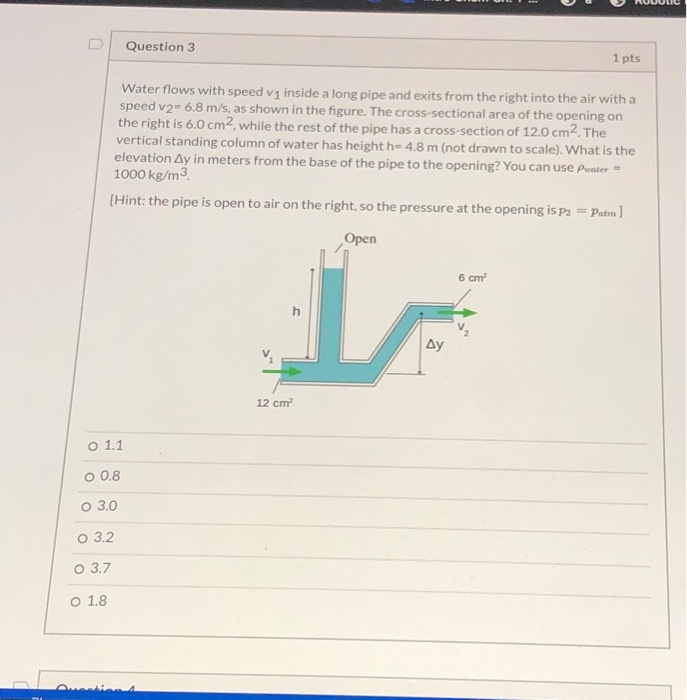Question 3 1 pts Water flows with speed v1 inside a long pipe and exits from the right into the air with a speed v2- 6.8 m/s, as shown in the figure. The cross-sectional area of the opening on the right is 6.0 cm2, while the rest of the pipe has a cross-section of 12.0 cm2. The vertical standing column of water has height h= 4,8 m (not drawn to scale). What is the elevation Ay in meters from the...

• ### A Venturi meter is a device for measuring the speed of a fluid within a pipe....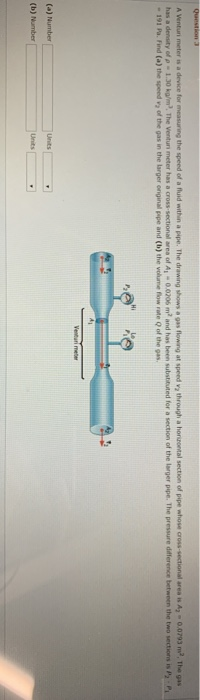A Venturi meter is a device for measuring the speed of a fluid within a pipe. The drawing shows a l lowing a speed through a horizontal section of pipe whose cross-sectional area is Az - 0.0793 m. The gas has a density of -1.Joka/m. The Ventun meter has a cross-sectional area of A - 0.0706m and has been substituted for a section of the larger pipe. The pressure difference between the two sections is P2P -191 PaFind (a) the...

• ### There is an oil of 730 kg/m3 density is streaming in an horizontal pipe of variable...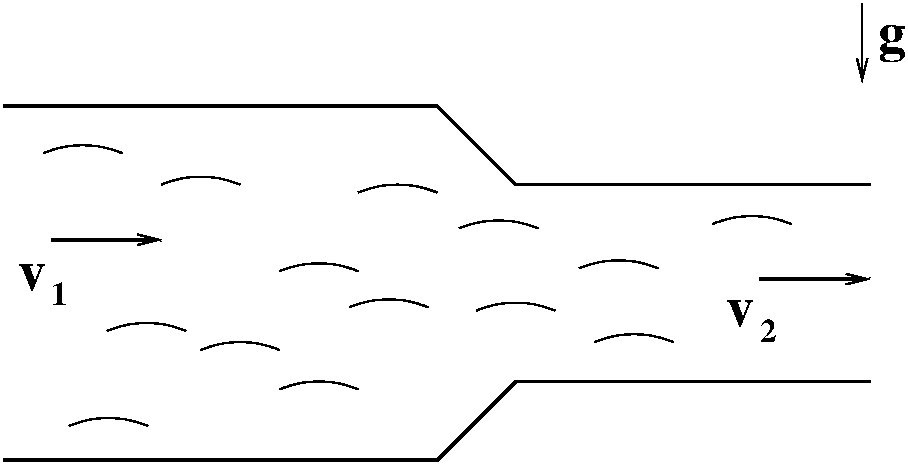There is an oil of 730 kg/m3 density is streaming in an horizontal pipe of variable cross area. The oil is siphoned through the pipe at a constant volume flow rate of 2.8 l/s. How long will it take to siphon 1700 kg of oil through the pipe? t = Unit in min The left‑end cross segment of the pipe has a range of 6.1 cm, what is the speed of the oil at which it streams trough this cross...

• ### In the figure, water flows through a horizontal pipe and then out into the atmosphere at...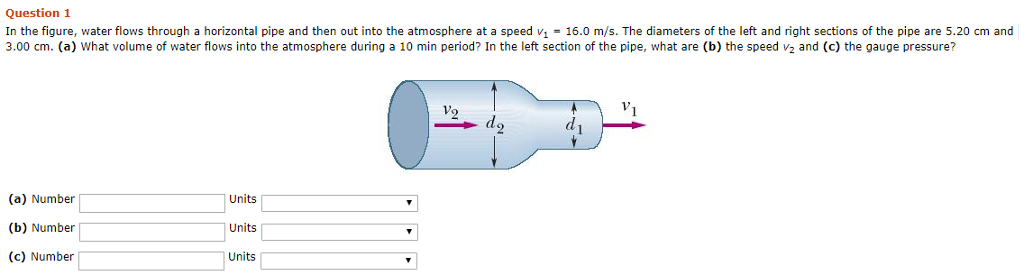In the figure, water flows through a horizontal pipe and then out into the atmosphere at a speed v1 = 16.0 m/s. The diameters of the left and right sections of the pipe are 5.20 cm and 3.00 cm. (a) What volume of water flows into the atmosphere during a 10 min period? In the left section of the pipe, what are (b) the speed v2 and (c) the gauge pressure? Question 1 In the figure, water flows through a...

• ### The figure below shows a horizontal pipe with a varying cross section. A liquid with a...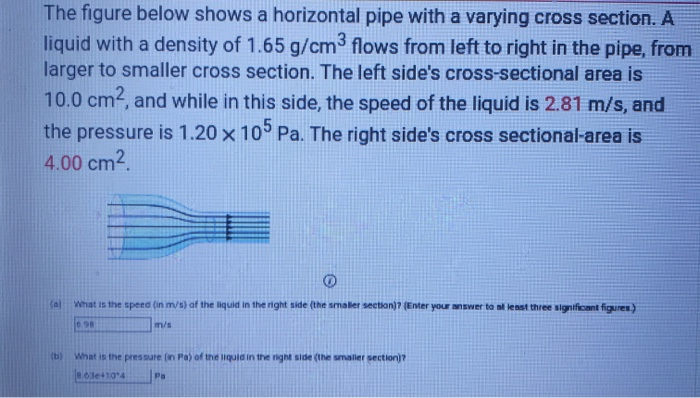The figure below shows a horizontal pipe with a varying cross section. A liquid with a density of 1.65 g/cm3 flows from left to right in the pipe, from larger to smaller cross section. The left side's cross-sectional area is 10.0 cm2, and while in this side, the speed of the liquid is 2.81 m/s, and the pressure is 1.20 x 10 Pa. The right side's cross sectional-area is 4.00 cm (Enter your answer to at least three significant figures...